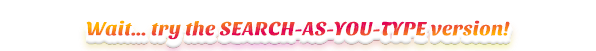# Summarize Data

Pandas provides a large set of summary functions that operate on different kinds of pandas objects (DataFrame columns, Series, GroupBy, Expanding and Rolling) and produce single values for each of the groups. When applied to a DataFrame, the result is returned as a pandas Series for each column.

## Basic descriptive statistics for numeric columns

# count, mean, std, min, max, percentiles
df.describe()


## Basic descriptive statistics for “object” columns (e.g. strings or timestamps)

# count, unique, top, and freq
df.describe(include=['object'])


## Basic descriptive statistics for all columns

df.describe(include='all')


## Basic descriptive statistics for only one column

df.column_x.describe()
# or if column name has spaces
df['column x'].describe()


## Count the number of occurrences of each value (excludes missing values)

df.column_x.value_counts()


## Count the number of occurrences of each value (includes missing values)

df.column_x.value_counts(dropna=False)


## Show the 3 most frequent occurances of column_x

df.column_x.value_counts()[0:3]


## Count number of rows in a DataFrame

# quicker
len(df.index)
# or
len(df)
# or
df.shape


## Count number of distinct values in a column

df.column_x.nunique()


## Get distinct values in a column

df.column_x.unique()


## Randomly select 30% of rows without replacement

df.sample(frac=0.3)


## Randomly select 30% of rows with replacement

df.sample(frac=0.3, replace=True)


## Randomly select 10 rows

df.sample(n=10)


## Randomly split a DataFrame into train/test

# will contain 75% of the rows
df_train = df.sample(frac=0.75)
# will contain the other 25% of rows
df_test = df[~df.index.isin(df_train.index)]


## Get first 7 rows ordered by the given columns in descending order

# better performance
df.nlargest(7, ['column_x', 'column_y'])
# equivalent with
df.sort_values(['column_x', 'column_y'], ascending=False).head(7)


## Get first 7 rows ordered by the given columns in ascending order

# better performance
df.nsmallest(7, ['column_x', 'column_y'])
# equivalent with
df.sort_values(['column_x', 'column_y'], ascending=True).head(7)